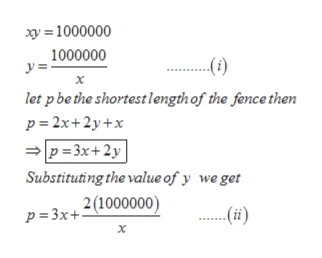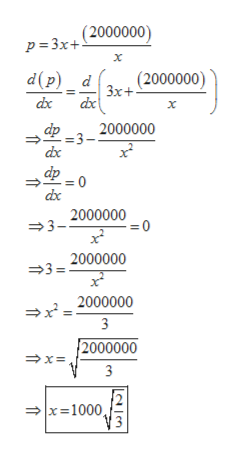# A rancher wants to fence in an area of 1000000 square feet in a rectangular field and then divide it in half with a fence down the middle parallel to one side. What is the shortest length of fence that the rancher can use? Note: The answer to this problem requires that you enter the correct units. (Enter your answer with a space before the units)

Question
70 views

A rancher wants to fence in an area of 1000000 square feet in a rectangular field and then divide it in half with a fence down the middle parallel to one side. What is the shortest length of fence that the rancher can use? Note: The answer to this problem requires that you enter the correct units. (Enter your answer with a space before the units)

check_circle

Step 1

A rancher wants to fence in an area of 1000000 square feet in a rectangular field and then divide it in half with a fence down the middle parallel to one side. What is the shortest length of fence that the rancher can use? Note: The answer to this problem requires that you enter the correct units. (Enter your answer with a space before the units)

Step 2

Let the dimensions of the field be x and yhelp_outlineImage Transcriptionclosexy = 1000000 1000000 .(i) х let p be the shortestlengthof the fence then p= 2x+2y+x =p= 3x+2y| Substituting the value of y we get 2(1000000) p = 3x+: ..... fullscreen
Step 3

To maximize the function find the  fi...help_outlineImage Transcriptionclose(2000000) p= 3x+ х (2000000) 3x+ d(p) _ d dx dx х dp 2000000 =3– dx фp dx 2000000 =3- 2000000 =3=. 2000000 3 {2000000 3 x=1000, = fullscreen

### Want to see the full answer?

See Solution

#### Want to see this answer and more?

Solutions are written by subject experts who are available 24/7. Questions are typically answered within 1 hour.*

See Solution
*Response times may vary by subject and question.
Tagged in
MathCalculus

### Derivative